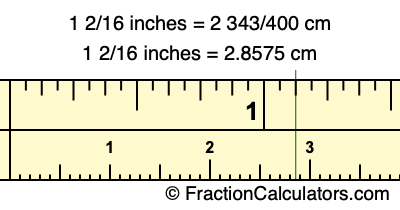Convert 1 2/16 inches to cmHere is how to convert 1 2/16 inches to cm. We will give you the fractional answer, the decimal answer, and illustrate the answer on our tape measure.

One inch is equal to 127/50 centimeters. Therefore, to get the fractional answer to 1 2/16 inches to cm, we multiply 1 2/16 inches by 127/50 and simplify if necessary.

inches × 127/50 = cm
1 2/16 × 127/50 = cm
1 2/16 × 127/50 = 2 343/400
1 2/16 inches = 2 343/400 cm

Centimeters is usually given in decimal form, so we have also converted the fractional answer to 1 2/16 inches to cm, to decimal form:

2 343/400 = 2.8575
1 2/16 inches = 2.8575 cm

It is sometimes hard to find where a fractional measurement is on a tape measure. Our tape measure image below shows exactly where 1 2/16 inches is located, so you know exactly where to find it.The top row of the tape measure is inches, and the bottom row is centimeters. As you can see, we marked 1 2/16 inches with a green line, so you can see where it is in relation to centimeters.

Inches to Centimeters Calculator
Here you can convert another length in inches to centimeters.

Convert   / inches to cm

Convert 1 3/24 inches to cm
Here is another inches to centimeters calculation we did for you.